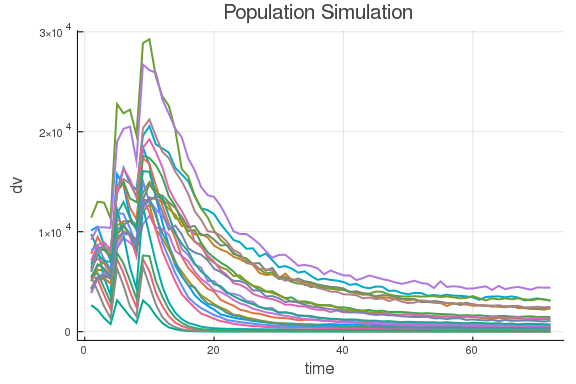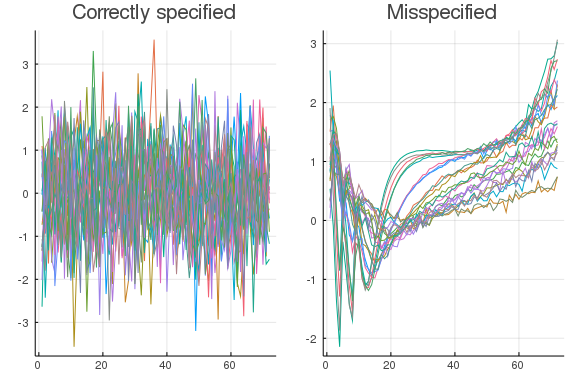# Fitting a PK model

In this tutorial we will go through the steps required to fit a model in Pumas.jl.

## The dosage regimen

We start by simulating a population from a two compartment model with oral absorption, and then we show how to fit and do some model validation using the fit output.

using Pumas, Plots, Random
Random.seed!(0);


The dosage regimen is an dose of 100 into Depot at time 0, followed by two additional (addl=2) doses every fourth hour

repeated_dose_regimen = DosageRegimen(100, time=0, ii=4, addl=2)

Pumas.DosageRegimen(1×9 DataFrames.DataFrame. Omitted printing of 2 columns
│ Row │ time    │ cmt   │ amt     │ evid │ ii      │ addl  │ rate    │
│     │ Float64 │ Int64 │ Float64 │ Int8 │ Float64 │ Int64 │ Float64 │
├─────┼─────────┼───────┼─────────┼──────┼─────────┼───────┼─────────┤
│ 1   │ 0.0     │ 1     │ 100.0   │ 1    │ 4.0     │ 2     │ 0.0     │)


As ususal, let's define a function to choose body weight randomly per subject

choose_covariates() = (Wt = rand(55:80),)

choose_covariates (generic function with 1 method)


and generate a population of subjects with a random weight generated from the covariate function above

pop = Population(map(i -> Subject(id = i,
evs = repeated_dose_regimen,
obs=(dv=Float64[],),
cvs = choose_covariates()),
1:24))

Population
Subjects: 24
Covariates: Wt
Observables: dv


We now have 24 subjects equipped with a weight and a dosage regimen.

## The PK model of drug concentration and elimination

To simulate a data set and attempt to estimate the data generating parameters, we have to set up the actual pharmacokinetics (PK) model and simulate the data. We use the closed form model called Depots1Central1Periph1 which is a two compartment model with first order absorption. This requires CL, Vc, Ka, Vp, and Q to be defined in the @pre-block, since they define the rates of transfer between (and out of) the compartments

mymodel = @model begin
@param   begin
cl ∈ RealDomain(lower = 0.0, init = 1.0)
tv ∈ RealDomain(lower = 0.0, init = 10.0)
ka ∈ RealDomain(lower = 0.0, init = 1.0)
q  ∈ RealDomain(lower = 0.0, init = 0.5)
Ω  ∈ PDiagDomain(init = [0.9,0.07, 0.05])
σ_prop ∈ RealDomain(lower = 0,init = 0.03)
end

@random begin
η ~ MvNormal(Ω)
end

@covariates Wt

@pre begin
CL = cl * (Wt/70)^0.75 * exp(η)
Vc = tv * (Wt/70) * exp(η)
Ka = ka * exp(η)
Vp = 30.0
Q  = q
end

@dynamics Depots1Central1Periph1

@derived begin
cp := @. 1000*(Central / Vc) # We use := because we don't want simobs to store the variable
dv ~ @. Normal(cp, abs(cp)*σ_prop)
end
end

PumasModel
Parameters: cl, tv, ka, q, Ω, σ_prop
Random effects: η
Covariates: Wt
Dynamical variables: Depot, Central, Peripheral
Derived: dv
Observed: dv


Some parameters are left free by giving them domains in the @param-block, and one PK parameter (the volume of distribution of the peripheral compartment) is fixed to 20.0.

## Simulating the indiviual observations

The simobs function is used to simulate individual time series. We input the model, the population of Subjects that currently only have dosage regimens and covariates, the parameter vector and the times where we want to simulate. Since we have a proportional error model we avoid observations at time zero to avoid degenerate distributions of the dependent variable. The problem is, that if the concentration is zero the variance in distribution of the explained variable will also be zero. Let's use the default parameters, as set in the @param-block, and simulate the data

param = init_param(mymodel)
obs = simobs(mymodel, pop, param, obstimes=1:1:72)
plot(obs)## Fitting the model

To fit the model, we use the fit function. It requires a model, a population, a named tuple of parameters and a likelihood approximation method.

result = fit(mymodel, Subject.(obs), param, Pumas.FOCEI())

FittedPumasModel

Successful minimization:                true

Likelihood approximation:        Pumas.FOCEI
Deviance:                          15184.132
Total number of observation records:    1728
Number of active observation records:   1728
Number of subjects:                       24

---------------------
Estimate
---------------------
cl          1.0153
tv          9.6367
ka          1.0528
q           0.49928
Ω₁,₁        1.0641
Ω₂,₂        0.057844
Ω₃,₃        0.029376
σ_prop      0.030778
---------------------


Of course, we started the fitting at the true parameters, so let us define our own starting parameters, and fit based on those values

alternative_param = (
cl = 0.5,
tv = 9.0,
ka = 1.3,
q  = 0.3,
Ω  = Diagonal([0.18,0.04, 0.03]),
σ_prop = 0.04)


FittedPumasModel

Successful minimization:                true

Likelihood approximation:        Pumas.FOCEI
Deviance:                          15184.132
Total number of observation records:    1728
Number of active observation records:   1728
Number of subjects:                       24

---------------------
Estimate
---------------------
cl          1.0152
tv          9.6367
ka          1.0528
q           0.49928
Ω₁,₁        1.0641
Ω₂,₂        0.057843
Ω₃,₃        0.029374
σ_prop      0.030778
---------------------


and we see that the estimates are essentially the same up to numerical noise.

To augment the basic information listed when we print the results, we can use infer to provide RSEs and confidence intervals

infer(result)

Calculating: variance-covariance matrix. Done.
FittedPumasModelInference

Successful minimization:                true

Likelihood approximation:        Pumas.FOCEI
Deviance:                          15184.132
Total number of observation records:    1728
Number of active observation records:   1728
Number of subjects:                       24

---------------------------------------------------------------
Estimate         RSE                  95.0% C.I.
---------------------------------------------------------------
cl         1.0153       21.071           [0.596   ;  1.4346  ]
tv         9.6367        4.8981          [8.7116  ; 10.562   ]
ka         1.0528        3.5841          [0.97883 ;  1.1267  ]
q          0.49928       0.21014         [0.49722 ;  0.50133 ]
Ω₁,₁       1.0641       24.937           [0.54401 ;  1.5842  ]
Ω₂,₂       0.057844     21.901           [0.033014;  0.082674]
Ω₃,₃       0.029376     27.784           [0.013379;  0.045372]
σ_prop     0.030778      1.6762          [0.029767;  0.031789]
---------------------------------------------------------------


So as we observed earlier, the parameters look like they have sensible values. The confidence intervals are a bit wide, and especially so for the random effect variability parameters. To see how we can use simulation to better understand the statistical properties of our model, we can simulate a much larger population and try again

pop_big = Population(map(i -> Subject(id = i,
evs = repeated_dose_regimen,
obs=(dv=Float64[],),
cvs = choose_covariates()),
1:100))
obs_big = simobs(mymodel, pop_big, param, obstimes=1:1:72)
result_big = fit(mymodel, read_pumas(DataFrame(obs_big); cvs=[:Wt]), param, Pumas.FOCEI())
infer(result_big)

Calculating: variance-covariance matrix. Done.
FittedPumasModelInference

Successful minimization:                true

Likelihood approximation:        Pumas.FOCEI
Deviance:                          64794.606
Total number of observation records:    7200
Number of active observation records:   7200
Number of subjects:                      100

-----------------------------------------------------------------
Estimate          RSE                   95.0% C.I.
-----------------------------------------------------------------
cl         0.94609        9.5559           [0.76889 ;  1.1233  ]
tv         9.8081         2.4371           [9.3396  ; 10.277   ]
ka         1.02           2.2383           [0.97528 ;  1.0648  ]
q          0.49991        0.097131         [0.49895 ;  0.50086 ]
Ω₁,₁       0.91311       12.385            [0.69146 ;  1.1348  ]
Ω₂,₂       0.058401      14.82             [0.041437;  0.075364]
Ω₃,₃       0.046506      15.246            [0.032609;  0.060402]
σ_prop     0.029836       0.80959          [0.029362;  0.030309]
-----------------------------------------------------------------


This time we see similar estimates, but much narrower confidence intervals across the board.

## Estimating a misspecified model

To explore some of the diagnostics tools available in Julia, we can try to set up a model that does not fit out data generating process. This time we propose a one compartent model. The problem with estimating a one compartment model when the data comes from a two compartment model, is that we cannot capture the change in slope on the concentration profile you get with a two compartment model. This means that even if we can capture the model fit someone well on average, we should expect to see systematic trends in the residual diagnostics post estimation.

mymodel_misspec = @model begin
@param   begin
cl ∈ RealDomain(lower = 0.0, init = 1.0)
tv ∈ RealDomain(lower = 0.0, init = 20.0)
ka ∈ RealDomain(lower = 0.0, init = 1.0)
Ω  ∈ PDiagDomain(init = [0.12, 0.05, 0.08])
σ_prop ∈ RealDomain(lower = 0, init = 0.03)
end

@random begin
η ~ MvNormal(Ω)
end

@pre begin
CL = cl * (Wt/70)^0.75 * exp(η)
V = tv * (Wt/70) * exp(η)
Ka = ka * exp(η)
end
@covariates Wt

@dynamics Depots1Central1

@derived begin
cp = @. 1000*(Central / V)
dv ~ @. Normal(cp, abs(cp)*σ_prop)
end
end

PumasModel
Parameters: cl, tv, ka, Ω, σ_prop
Random effects: η
Covariates: Wt
Dynamical variables: Depot, Central
Derived: cp, dv
Observed: cp, dv

result_misspec = fit(mymodel_misspec, read_pumas(DataFrame(obs); cvs=[:Wt]), alternative_param, Pumas.FOCEI())

FittedPumasModel

Successful minimization:                true

Likelihood approximation:        Pumas.FOCEI
Deviance:                          25495.562
Total number of observation records:    1728
Number of active observation records:   1728
Number of subjects:                       24

---------------------
Estimate
---------------------
cl          1.3637
tv         25.674
ka     458600.0
Ω₁,₁        0.48383
Ω₂,₂        0.13698
Ω₃,₃        0.048852
σ_prop      0.52776
---------------------


First off, the absorption flow parameters ka is quite off the charts, so that would be a warning sign off the bat, but let us try to use a tool in the toolbox to asses the fit: the weighted residuals. We get these by using the wresiduals function

wres = wresiduals(result)
wres_misspec = wresiduals(result_misspec)
p1 = plot([w.wres.dv for w in wres], title="Correctly specified", legend=false)
p2 = plot([w.wres.dv for w in wres_misspec], title = "Misspecified", legend=false)
plot(p1, p2)The weighted residuals should be standard normally distributed with throughout the time domain. We see that this is the case for the correctly specified model, but certainly not for the misspecified model. That latter has a very clear pattern in time. This comes from the fact that the one compartment model is not able to capture the change in slope as time progresses, so it can never accurately capture the curves generated by a two compartment model.

### Conclusion

This tutorial showed how to use fitting in Pumas.jl based on a simulated data set. There are many more models and simulation experiments to explore. Please try out fit on your own data and model, and reach out if further questions or problems come up.